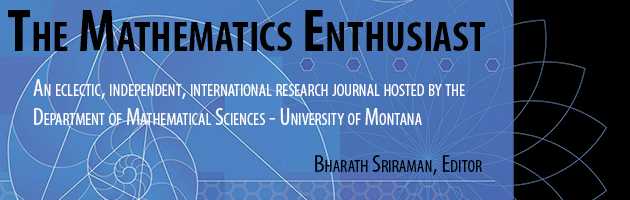•
•#### Article Title

Two-step arithmetic word problems

10

1-2

#### Abstract

This study uses the perspective of schemes to analyze characteristics of arithmetic word problems that can influence the process of translation from the verbal statement to an arithmetical representation. One characteristic that we have detected in the two-step word problems is the presence of one or two connections (nodes) in schemes that represent them, and this paper explores whether the number of nodes affects the activation of the associated schemas. With students from the 5th and 6th grades of elementary school (11 and 12 years of age), we analyze the written productions and would stress that the number of connections influences the activation of the right schema. Results show that the double connection implicate a greater difficulty for obtaining a correct arithmetical representation. Likewise, the presence of a simple or double connection between the two relationships means that the students commit specific errors that we associate with this characteristic.

379

406

#### Digital Object Identifier (DOI)

10.54870/1551-3440.1272

COinS